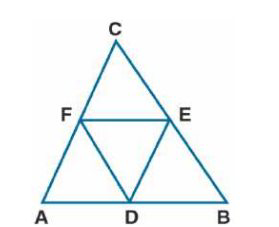# ML Aggarwal Solutions Class 9 Mathematics Solutions for Mid Point Theorem Exercise 11 in Chapter 11 - Mid Point Theorem

Prove that the four triangles formed by joining in pairs the mid-points of the sides C of a triangle are

congruent to each other

It is given that

In Δ ABC

D, E, and F are the mid-points of AB, BC, and CA

Now join DE, EF, and FD

To find:

Δ ADF ≅ Δ DBE ≅ Δ ECF ≅ Δ DEFTo prove:

In Δ ABC

D and E are the mid-points of AB and BC

DE || AC or FC

Similarly DF || EC

DECF is a parallelogram

We know that

Diagonal FE divides the parallelogram DECF in two congruent triangles DEF and CEF

Δ DEF ≅ Δ ECF …… (1)

Here we can prove that

Δ DBE ≅ Δ DEF …. (2)

Δ DEF ≅ Δ ADF ……. (3)

Using equation (1), (2) and (3)

Δ ADF ≅ Δ DBE ≅ Δ ECF ≅ Δ DEF

Video transcript
Related Questions
Exercises

Lido

Courses

Teachers

Book a Demo with us

Syllabus

Maths
CBSE
Maths
ICSE
Science
CBSE

Science
ICSE
English
CBSE
English
ICSE
Coding

Terms & Policies

Selina Question Bank

Maths
Physics
Biology

Allied Question Bank

Chemistry
Connect with us on social media!